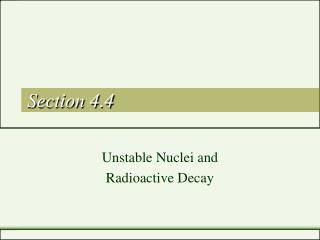DownloadDownload PresentationSection 4.4

# Section 4.4

Download Presentation## Section 4.4

- - - - - - - - - - - - - - - - - - - - - - - - - - - E N D - - - - - - - - - - - - - - - - - - - - - - - - - - -
##### Presentation Transcript

1. Section 4.4 Unstable Nuclei and Radioactive Decay

2. Objectives • Explain the relationship between unstable nuclei and radioactive decay. • Characterize alpha, beta, and gamma radiation in terms of mass and charge.

3. Radioactivity • Chemical Reactions • Atoms of one element do not change into atoms of another element during a chemical reaction. • Why?

4. Radioactivity • Chemical reactions involve the rearrangement of atoms – not their “destruction.” • Chemical reactions involve only an atom’s electrons – the nucleus remains unchanged.

5. Radioactivity • Nuclear Reactions • These are reactions that involve an atom of one element changing into an atom of another element. These reactions involve the change of an atom’s nucleus.

6. Radioactivity • In the late 1890’s, radioactivity was discovered. • Radioactivity is a process in which some substances spontaneously emit radiation. • Radiation is the name for the energy (“rays”) and particles emitted by radioactive material. • Scientists discovered that by emitting radiation, atoms of one element could change into atoms of another element.

7. Radioactivity • Why does radioactivity occur? • Radioactive atoms emit radiation because their nuclei are unstable*. • Unstable systems gain stability by losing energy. • Unstable nuclei lose energy by emitting radiation in a spontaneous process called radioactive decay. • Radioactive decay continues until stable, non-radioactive atoms form. They are often of a different element.

8. Types of Radiation • Research, begun in the late 1880’s, used electrically charged plates. Scientists were able to identify 3 types of radiation.

9. Types of Radiation • Alpha Radiation • Deflected to negatively charged plates. • Made up of alpha particles: 2 protons and 2 neutrons with a +2 charge. • Symbol for an alpha particle is 42αor 42He (the helium nucleus) • Example of alpha decay

10. Alpha Radiation • Note what happens to the atomic number of an element upon emission of an alpha particle. • The atom giving up the alpha particle has its atomic number reduced by two. This results in the atom becoming a different element. In the example, Radium-226 undergoes alpha decay to form Radon-222.

11. Alpha Radiation 22688Ra --> 22286Rn + 42He • This type of equation is known as a nuclear equation. • The atomic numbers and mass numbers of all particles involved are shown. • Note that both atomic number and mass number are conserved in a nuclear equation.

12. Practice Problems Complete the following nuclear equations for alpha decay: • 24195Am ----> • _____ ----> 42He + 23592U • 23592U ----> • _____ ----> 42He + 20684Po

13. Types of Radiation • Beta radiation • Deflected towards the positively charged plate • Consists of fast-moving electrons called beta particles. They have a 1- charge. • Symbol for a beta particle is 0-1ß or 0-1e. • Example of beta decay

14. Beta Radiation • The atom giving up the beta particle has its atomic number increased by one. • This results in the atom becoming a different element. In the example, carbon-14 undergoes beta decay to form nitrogen-14, according to the equation: 146C ---> 0-1e + 147N. • Note that, in the equation, atomic numbers and mass numbers are conserved.

15. Beta Radiation • Since beta radiation is a type of nuclear radiation, the beta particle is emitted by the nucleus of an atom. • How, you may ask, is it possible for an electron to come from a nucleus?? • Scientists believe that neutrons are actually composed of 2 particles - an electron and a proton. • In beta decay, the electron is emitted and the proton is left, increasing the atomic number.

16. Practice Problems Complete the following nuclear equations for beta decay. 1. 6027Co ----> 2. _____ ---> 0-1e + 23191Pa 3. 9740Zr ---> 4. _____ ---> 0-1e + 6630Zn

17. Types of Radiation • Gamma Radiation • Also called gamma rays • It is high-energy electromagnetic radiation. It has no charge. It also has no mass so its emission does not change the element. • However, gamma radiation often accompanies alpha and beta emission, which do change the element's identity. • Symbol for a gamma ray is 00 ϒ or just ϒ • Gamma rays account for most of the energy lost during the radioactive decay process.